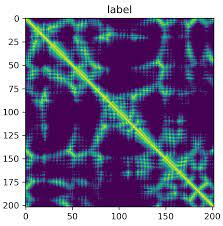# Use case: distance matrix -> 3d protein (biology)

This is another “proof of concept” post.

Background: In the first Alphafold model (v1) from Deep Mind (2018) they used a model which output a matrix of distances from each amino-acid to each other which they call a “distogram”. They then had to use this matrix to reconstruct the protein. Here is Demis talking about the distogram:

Here is what one looks like:(*In actual fact the model outputs a set of probabilities for the distances in the distogram but we will just use the most probable distances for simplicity - but it will be less accurate as a result).

Now there is a torch implementation of Alphafold v1. But doesn’t seem to include the final step of reconstructing the 3D molecule from the “distogram”. But Demis does mention somewhere that they did this using gradient descent… thus it could be done using Sentis in Unity.

I thought it might be interesting to try to reconstruct this final step. (This is not the step which predicts the shape of the protein but just the step which reconstructs the 3d shape from the output).

Making the model
We need a loss function which is lowest when the predicted distances match the distances in the distogram, D_{ij}, and we assume that the shorter distances are more accurate than bigger ones. The function E =\sum\limits_{ij}\left( 1 - \frac{|x_i - x_j|^2}{(D_{ij})^2+\varepsilon} \right)^2 seems to work fairly well as a starting point. We can create a model in pytorch by calculating model(x,D) = \partial E/\partial x_i with a bit of high school math(s) to create a model where the inputs are x and D and the output is \delta x. Then we export this model to ONNX. Each frame we run the model and update the positions of the amino acids using x\rightarrow x+\delta x.
Visualisation
For simplicity we just represent the amino acids by spheres linked by cylinders.

For better visualisation there may be some unity projects like UnityMol.

Comparison
Here we take the predicted distogram predicted by alphafold-torch model and reconstructed in Unity Sentis and compare it to the actual protein. (This is using an online viewer to render the proteins from a coordinate file):

We see it’s reconstructed the main features such as the main horizontal alpha-helix spiral (green). The reason why it’s not 100 accurate is more to do with the Alphafold model v1 not being as accurate as v2. There’s probably some more issues such as selected the best loss function etc. It at least gives a better idea of what the structure would look like compared to the distogram.

In contrast here is the same model using a distogram from a fake-protein. It works faster since the distogram is more accurate and not just a guess from another model.

Why is this interesting?
The newer protein folding models (e.g. Alphafold2, Omegafold, ESMfold (from Meta) etc.) don’t use a “distogram” as they output the 3d coordinates directly. But I think it is still interesting as it shows generally how neural networks can be used to model large clusters of agents whether they be atoms, birds, or alien hoards.

This is actually a “train-on-device” example in disguise in which we only have one piece of data which is the distrogram which we want to train our model to fit it.

Future
It may be nice to implement one of the newer models e.g. Omegafold fully in Unity to get end-to-end protein folding. (An advantage would be it could run on any device and not need to worry about python versioning etc. Maybe good for distributive computing?) Some of the newer models work like language models by remembering similar patterns of chains of amino-acids to predict how it will fold up.

3 Likes

Let me just add some extra references which I found useful as I try to implement Alphafold v1 in unity:

A fairly detailed talk:

It takes about <1 second for each 64x64 window to run the model in Unity. The model is supposed to be run multiple times as it scans over the sequence. Then you add the outputs to get the distogram. The only difficult bit is constructing the input which involves quite a lot of features. So far I’ve got it working using the saved inputs from the example. Which proves at least the neural net part of it works.

1 Like

Thanks for sharing! This is insane!